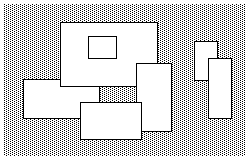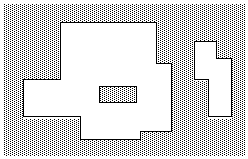## Picture

A number of rectangular posters, photographs and other pictures of the same shape are pasted on a wall. Their sides are all vertical or horizontal. Each rectangle can be partially or totally covered by the others. The length of the boundary of the union of all rectangles is called the perimeter.

Write a program to calculate the perimeter.

An example with 7 rectangles is shown in Figure 1.Figure 1. A set of 7 rectangles

The corresponding boundary is the whole set of line segments drawn in Figure 2.Figure 2. The boundary of the set of rectangles

The vertices of all rectangles have integer coordinates.

### Input Format

The first line of the input contains the number of rectangles (0 ≤ number of rectangles < 5000) pasted on the wall. In each of the subsequent lines, one can find the integer coordinates of the lower left vertex and the upper right vertex of each rectangle. The values of those coordinates are given as ordered pairs consisting of an x-coordinate followed by a y-coordinate. All coordinates are in the inclusive range [−10000, 10000] and any existing rectangle has a positive area.

### Output Format

The output must contain a single line with a non-negative integer which corresponds to the perimeter for the input rectangles. The numeric value of the result may need a 32-bit signed representation.

### Sample Input

```7
-15 0 5 10
-5 8 20 25
15 -4 24 14
0 -6 16 4
2 15 10 22
30 10 36 20
34 0 40 16```

This corresponds to the example of Figure 1.

### Sample Output

`228`

Point Value: 20
Time Limit: 2.00s
Memory Limit: 32M